# How to use the SUMPRODUCT function in Google SheetsThe SUMPRODUCT function in Google Sheets multiplies corresponding items within arrays of equal size and returns the sum of the results. This is useful in many situations where you need to multiply items across arrays and add them up.

Take a look at the example below. Here I’ve got a list of items, their prices, quantities, and total sale prices.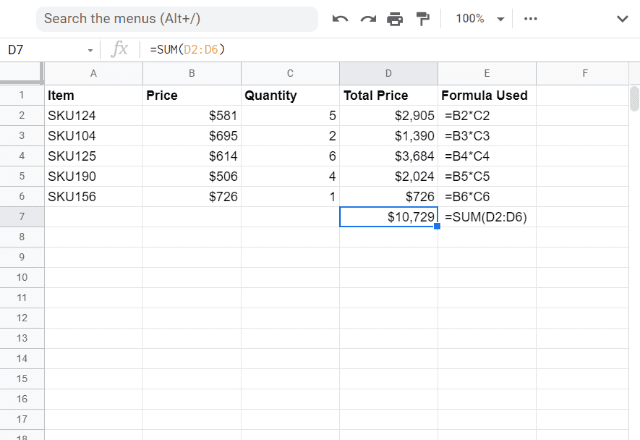I calculated the corresponding sale prices in column D, i.e. cells D2 through to D6.

To do this, I used multiplication functions to multiply each price by its quantity.

Then, I used the SUM function to calculate the total sales price across all the items (cell D7).

This is a rather lengthy process. Fortunately, there’s a much simpler alternative: the SUMPRODUCT function.

#### Syntax

=SUMPRODUCT(array1, [array2, …])

• array1 – the array or address reference for the range of cells whose values the SUMPRODUCT function will multiply with corresponding values in the second array or range of cells.
• array2, … – optional and additional arrays or address references to the range of cells with the same size as array1. The SUMPRODUCT function will multiply these values with corresponding values in the first array or range of cells.

### How to use the SUMPRODUCT function

In the example above, I used the SUM function to get the results I wanted.

Here’s how to carry out the same calculation using the more efficient SUMPRODUCT function.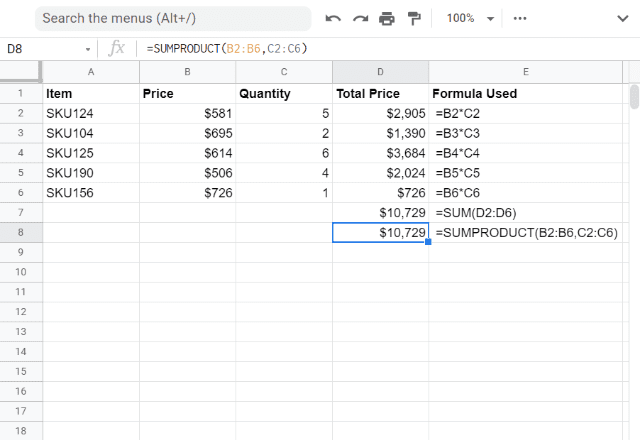In the snapshot above, I keyed in the SUMPRODUCT function into cell D8 by typing =SUMPRODUCT(B2:B6,C2:C6).

Compare the value it returned with that in cell D7: it’s exactly the same.

The range B2:B6 is the input for array1, while C2:C6 is the input for array2. SUMPRODUCT multiplies and adds up the values all in one step – much easier!

While this is already much handier than the former approach, it gets even better when the source data set is altered, especially when you insert new rows.

I’ll now try inserting a new record between the 4th and 5th rows: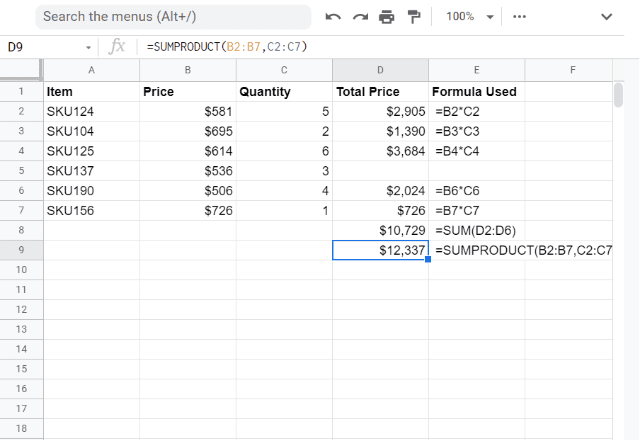You will notice that the SUMPRODUCT function is now relocated to cell D9. It automatically adjusted the calculation for the latest modification, while the SUM function did not change.

If you were to make the SUM function work, you would need to update the function in D5 and repeat this every time you add a new row, which could quickly become a time-consuming job.

#### SUMPRODUCT with multiple arrays

So far I’ve used the SUMPRODUCT function to multiply and add up two arrays. But you can use the SUMPRODUCT function with as many arrays as you want!

In the example below I’ve added a third column that shows the % commission paid for each product sold.

Using the SUMPRODUCT function, I can quickly multiply all three columns together for each item, then add up the total. I’ve done this by typing =sumproduct(B2:B6,C2:C6,D2:D6).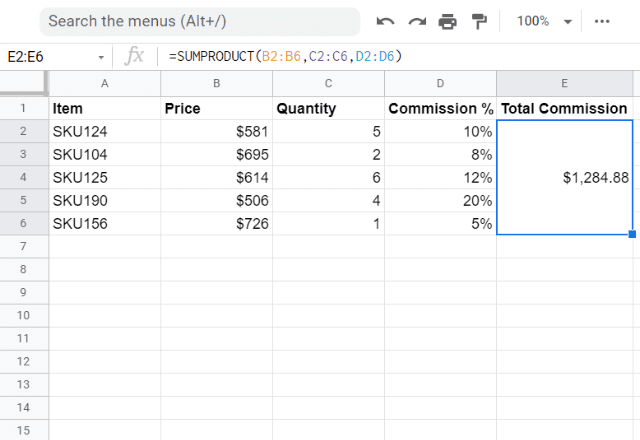#### Using SUMPRODUCT with conditions

Interestingly, you can also use the SUMPRODUCT function to sum items that meet certain criteria. This is similar to the COUNTIFS function.

I’ll demonstrate that in an example below. The function counts (or rather sums) the instances where all the array conditions evaluate to TRUE.

Here, I’m asking for the number of items that meet the three following criteria:

1. Are vegetables.
2. Have 25 or more calories.
3. Contain more than 5g of carbs.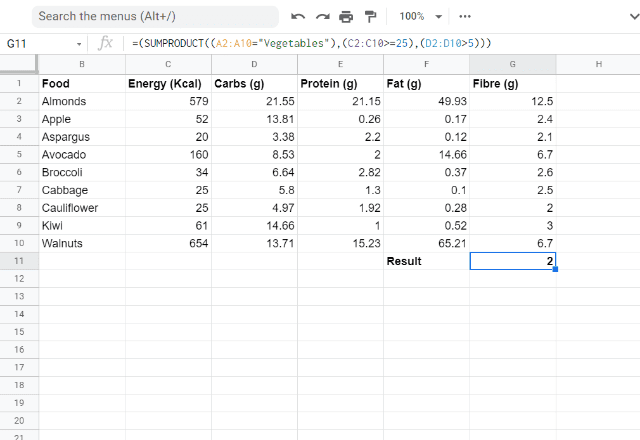As you can see, the SUMPRODUCT function searches the dataset and tells me that there are 2 items that meet these criteria.

The logic here may be confusing at first, but remember that in Google Sheets, True = 1 and False = 0. So when you use the SUMPRODUCT function with conditions that are True or False, you’re really just multiplying 1’s and 0’s. Of course, any False value (in other words, a 0) will make the entire entry 0. That’s why all the criteria must be true for the item to be counted.

To better illustrate this, I’ve included the table below.

Note that the product of TRUE, TRUE, and TRUE will be 1, whereas the product of any combination that involves a FALSE evaluates to 0.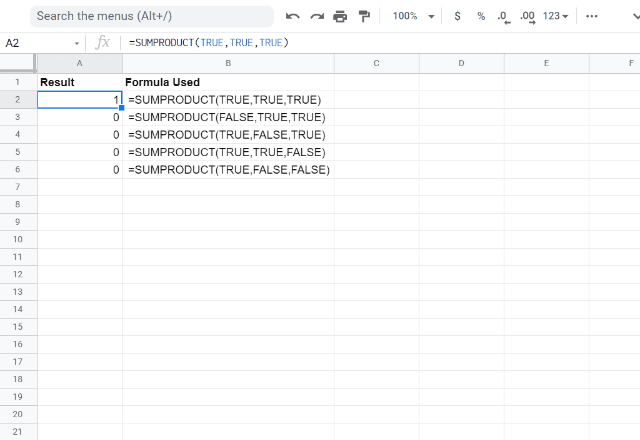Once you understand how SUMPRODUCT treats true and false values, you can experiment with more complex uses of the function.

You can use other functions to generate true or false for each item based on whatever criteria you want!

In the example below I’ll show you one way of doing that.

#### Complex SUMPRODUCT formulas

I’ll expand on the sales commission example. Let’s say that now, only items with an item number containing a 2 will receive commission. The commission for the other items has been waived.

To ask the function to calculate this, I’ve added a new column in which I use the ISNUMBER and SEARCH functions to determine whether there is a 2 in the item number (I do this by typing the formula =isnumber(search(2,A2))).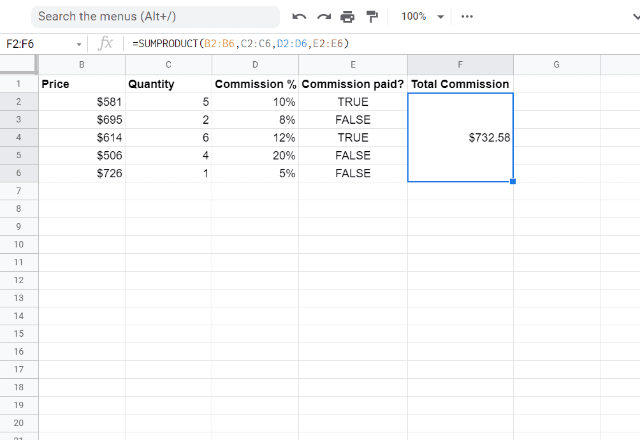Now I can do a SUMPRODUCT with 4 arrays, and the False values (i.e. 0’s) will eliminate the commission for the products without a 2 in their item number – leaving me with only the relevant products being included in the calculation.

As you can see, you can use the SUMPRODUCT function to accomplish both simple and complex tasks very efficiently!

#### Looking for more Google Sheets tips?

Check out our other formulas posts below!

Editor’s note: This is a revised version of a previous post that has been updated for accuracy and comprehensiveness.